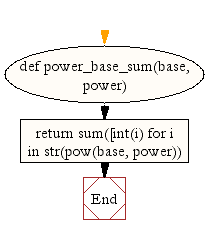﻿ Python Math: Calculate the sum of all digits of the base to the specified power - w3resource# Python Math: Calculate the sum of all digits of the base to the specified power

## Python Math: Exercise-12 with Solution

Write a Python program to calculate the sum of all digits of the base to the specified power.

Sample Solution:-

Python Code:

``````def power_base_sum(base, power):
return sum([int(i) for i in str(pow(base, power))])

print(power_base_sum(2, 100))
print(power_base_sum(8, 10))
```
```

Sample Output:

```115
37
```

Flowchart:## Visualize Python code execution:

The following tool visualize what the computer is doing step-by-step as it executes the said program:

Python Code Editor:

Have another way to solve this solution? Contribute your code (and comments) through Disqus.

What is the difficulty level of this exercise?

Test your Programming skills with w3resource's quiz.

﻿

## Python: Tips of the Day

Getting sorted iterable (can sort by "compare" function):

```>>> a = [1, 2, -3]
>>> sorted(a)
[-3, 1, 2]

>>> sorted(a,key=abs)
[1, 2, -3]
```